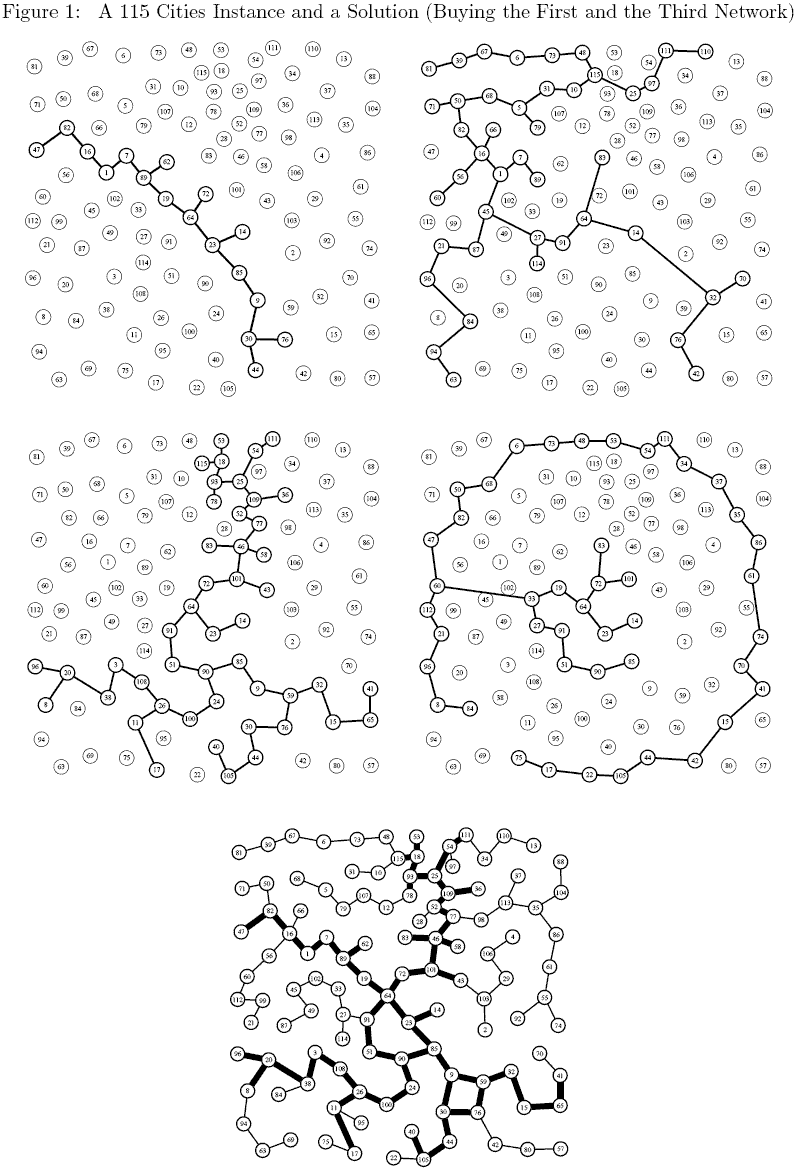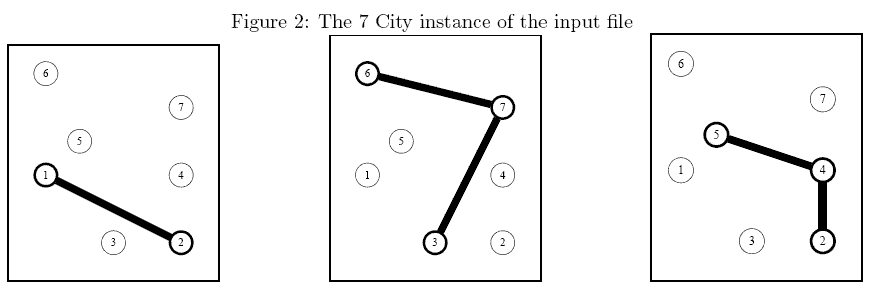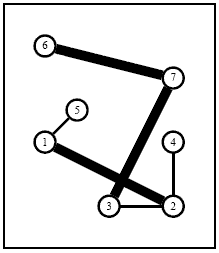Online JudgeProblem SetAuthorsOnline ContestsUser
Web Board
F.A.Qs
Statistical Charts
Problems
Submit Problem
Online Status
Prob.ID:
Register
Authors ranklist
Current Contest
Past Contests
Scheduled Contests
Award Contest
Register
Language:
 Time Limit: 2000MS Memory Limit: 65536K Total Submissions: 2428 Accepted: 836

Description

World Wide Networks (WWN) is a leading company that operates large telecommunication networks. WWN would like to setup a new network in Borduria, a nice country that recently managed to get rid of its military dictator Kurvi-Tasch and which is now seeking for investments of international companies (for a complete description of Borduria, have a look to the following Tintin albums ``King Ottokar's Sceptre", ``The Calculus Affair" and ``Tintin and the Picaros"). You are requested to help WWN todecide how to setup its network for a minimal total cost.
Problem
There are several local companies running small networks (called subnetworks in the following) that partially cover the n largest cities of Borduria. WWN would like to setup a network that connects all n cities. To achieve this, it can either build edges between cities from scratch or it can buy one or several subnetworks from local companies. You are requested to help WWN to decide how to setup its network for a minimal total cost.
• All n cities are located by their two-dimensional Cartesian coordinates.
• There are q existing subnetworks. If q>=1 then each subnetwork c ( 1<=c<=q ) is defined by a set of interconnected cities (the exact shape of a subnetwork is not relevant to our problem).
• A subnetwork c can be bought for a total cost wc and it cannot be split (i.e., the network cannot be fractioned).
• To connect two cities that are not connected through the subnetworks bought, WWN has to build an edge whose cost is exactly the square of the Euclidean distance between the cities.

You have to decide which existing networks you buy and which edges you setup so that the total cost is minimal. Note that the number of existing networks is always very small (typically smaller than 8).
A 115 Cities Instance
Consider a 115 cities instance of the problem with 4 subnetworks (the 4 first graphs in Figure 1). As mentioned earlier the exact shape of a subnetwork is not relevant still, to keep figures easy to read, we have assumed an arbitrary tree like structure for each subnetworks. The bottom network in Figure 1 corresponds to the solution in which the first and the third networks have been bought. Thin edges correspond to edges build from scratch while thick edges are those from one of the initial networks.

Input

The first line contains the number n of cities in the country ( 1<=n<=1000 ) followed by the number q of existing subnetworks ( 0<=q<=8 ). Cities are identified by a unique integer value ranging from 1 to n . The first line is followed by q lines (one per subnetwork), all of them following the same pattern: The first integer is the number of cities in the subnetwork. The second integer is the the cost of the subnetwork (not greater than 2 x 106 ). The remaining integers on the line (as many as the number of cities in the subnetwork) are the identifiers of the cities in the subnetwork. The last part of the file contains n lines that provide the coordinates of the cities (city 1 on the first line, city 2 on the second one, etc). Each line is made of 2 integer values (ranging from 0 to 3000) corresponding to the integer coordinates of the city.

Output

Your program has to write the optimal total cost to interconnect all cities.

Sample Input

```7 3
2 4 1 2
3 3 3 6 7
3 9 2 4 5
0 2
4 0
2 0
4 2
1 3
0 5
4 4```

Sample Output

```17
```

Hint

Sample Explanation: The above instance is shown in Figure 2. An optimal solution is described in Figure 3 (thick edges come from an existing network while thin edges have been setup from scratch).Figure 3: An optimal solution of the 7 City instance in which which the first and second existing networkshave been bought while two extra edges (1, 5) and (2, 4)Source

[Submit]   [Go Back]   [Status]   [Discuss]Home PageGo BackTo top